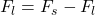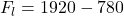## A diving board 3.00 m long is supported at a point 1.00 m from the end, and a diver weighing 500 N stands at the free end. The diving board

Question

A diving board 3.00 m long is supported at a point 1.00 m from the end, and a diver weighing 500 N stands at the free end. The diving board is of uniform cross section and weighs 280 N. Find:

a. the force at the support point
b. the force at the left-hand end.

in progress 0
2 months 2021-08-03T16:26:26+00:00 1 Answers 6 views 0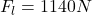Explanation:

From the question we are told that:

Length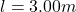Support distance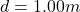Weight of diver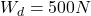Weight of board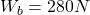Generally the equation for Total Torque is mathematically given by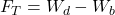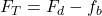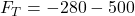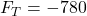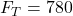Since we consider the horizontal axis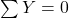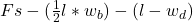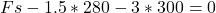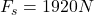Therefore  the force at the support point  1.00m from the force at the left-hand